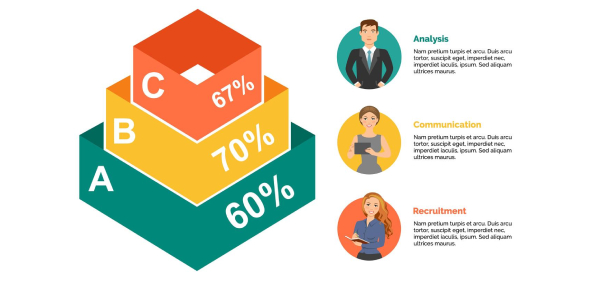# Percentage Quiz For Class 8

10 Questions | Attempts: 19011SettingsWhat do you know about percentages? Do you think you understand enough to pass this percentage quiz for class 8? A percentage is specifically a number telling you how many of something per hundred. To find a percent, divide the number of a portion of something by the total number possible. Then, you multiply by 100 and move the decimal two places to the right. Take this quiz to learn more about percentages.

• 1.
Sarah's bank account increased by 54%. If the bank account was \$600, it is now worth:
• A.

\$924

• B.

\$324

• C.

\$276

• D.

\$876

• 2.
What is 5% as a decimal?
• A.

0.5

• B.

5

• C.

0.005

• D.

0.05

• 3.
There are 321,200 people who played tennis last year, this year the number of players has decreased by 6%. How many people played tennis this year?
• A.

128,480

• B.

301,928

• C.

340,472

• D.

308,352

• 4.
What is 12.3 as a percentage?
• A.

1230%

• B.

12.3%

• C.

1.23%

• D.

123%

• 5.
16% of people have red hair. In a group of 300 people, how many would have red hair?
• A.

16

• B.

32

• C.

48

• D.

64

• 6.
What is 9% of 61?
• A.

54.9

• B.

9.31

• C.

4.96

• D.

5.49

• 7.
Julie got 98 out of 140 for a science exam. What percentage did she get?
• A.

98%

• B.

70%

• C.

68%

• D.

78%

• 8.
5 out of 8 students attended the Meeting. What percentage is this?
• A.

1.6%

• B.

160%

• C.

72%

• D.

62.5%

• 9.
Jordan lost 30% of his marbles. What is this as a Fraction in its simplest form?
• A.

30 / 100

• B.

3 / 10

• C.

30 / 1000

• D.

3 / 100

• 10.
What is 6.5% as a fraction, in its simplest form?
• A.

6.5 / 100

• B.

13 / 200

• C.

0.65 / 10

• D.

65 / 200

## Related TopicsBack to top
×

Wait!
Here's an interesting quiz for you.